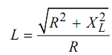## Quality Factor Assignment Help

Assignment Help: >> AC Circuits - Quality Factor

Quality Factor (Q-Factor):

Q-factor is the reciprocal of the power factor of coil. This is the figure of merit, that means. it must be as large as possible for any coil (combination of resistance and inductance).

Q = 2π  (Maximum energy stored /Energy dissipated per cycle)

or         Q = 1/ cos φ    = Z /RIf R is extremely less as compared to XL, then

Q = X L/ R = ωL/ R .